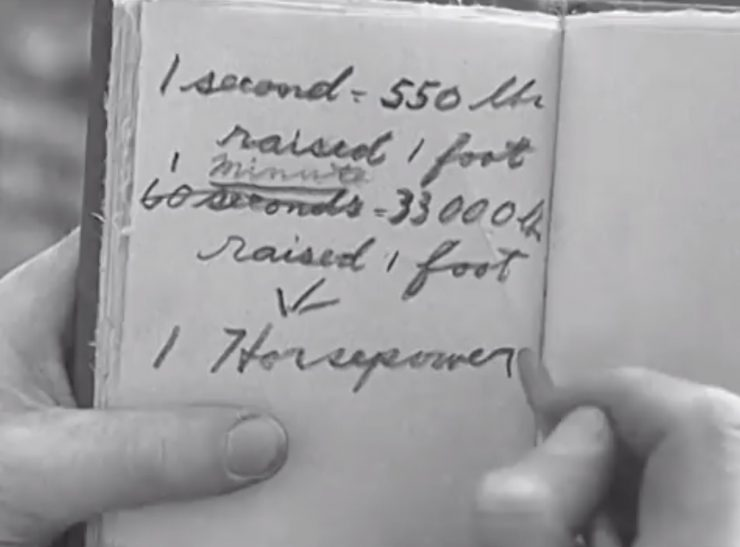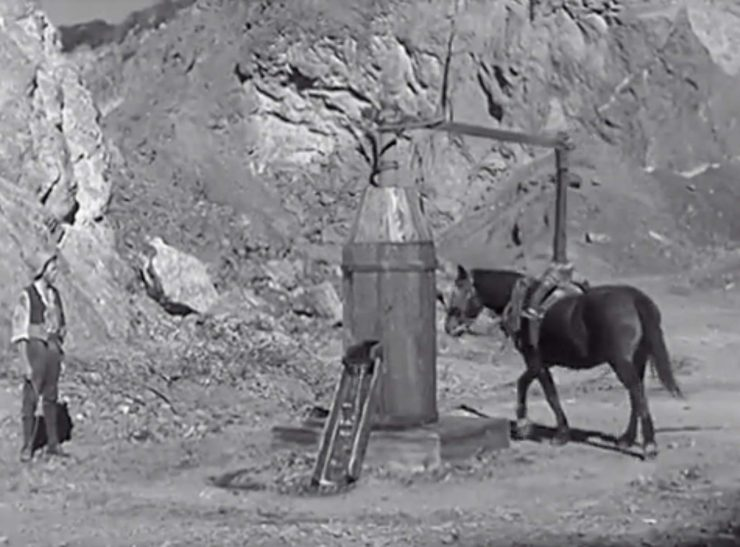We still frequently use the term horsepower as the primary indicator of how much power an engine produces, but very few can explain what it actually means and how it was originally calculated.

This concise 9 minute short film explains the origins of the term, how it was calculated by James Watt, and why it was used. Despite the fact that the film was originally produced in 1937 it’s still just as true today as it was then.

Some regions have been slowly moving away from using horsepower in favour of kilowatts, however it’s still far from catching on around the world, particularly with those of us over the age of 30.

To calculate what one horsepower is, James Watt studied working horses. He noted that the average horse under average conditions exerts the amount of power that would raise 550 lbs a distance of 1 foot in 1 second. Working continuously for one hour, the same power would move a total of 33,000 lbs a distance of 1 foot.

James Watt took this unit as a figure, and named it “one horsepower”.

One kilowatt is approximately 1.34 mechanical horsepower, and one horsepower is calculated as either an imperial horsepower with an output of 745.7 watts, or a metric horsepower at 735.5 watts.

If you’d like to read more about James Watt and the history of horsepower you can click here to visit the Encyclopaedia Brittanica entry.